Next: 2.7 The Fundamental Thermodynamic Relation Previous: 2.5 Entropy

# 2.6 Examples of entropy changes

Take-home message: Spontaneous changes are aways associated with entropy increase.

Below we have various examples of entropy change during various processes. From them we can draw some general conclusions. First we see in general the entropy of anything increases when it is heated, and the entropy of a gas increases when it expands at constant temperature. A common theme (to be explored in more detail later) is that an increase in entropy is associated with an increase in disorder.

Examples of spontaneous processes are the flow of heat from a hotter to a colder body (see Ex. 1) and the free expansion of a gas (see Ex. 4). In both of these, the total entropy increases (though that of parts of the system may decrease). This is completely general: spontaneous processes are those which increase entropy.

Since heat flow from hotter to colder bodies is irreversible, reversible processes must involve heat flow between bodies of the same temperature. It follows that any entropy change of the system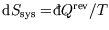must be exactly balanced by that of the heat bath which provided the heat: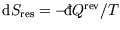. Thus the entropy change of the universe during reversible processes is zero.

During an adiabatic process no heat flows. Thus from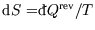we see that the entropy change of a system during for a reversible, adiabatic process is zero. But note that both qualifiers are needed; the entropy of a non-isolated system can change during a reversible process (and the entropy change of the surroundings will compensate), and an irreversible change to an isolated system will increase the entropy (see Ex. 1&4).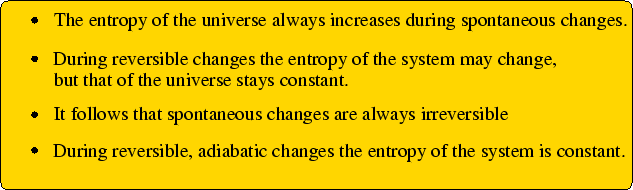Try these problems for yourself before checking the detailed answers!

• Ex. 1 Two identical blocks of iron, one at 100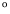C and the other at 0C, are brought into thermal contact. What happens? What is the total entropy change? (Assume the heat capacity of each block,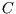, is constant over this temperature range, and neglect volume changes)

Answer: Both blocks end up at 50C and the entropy change is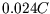. More details here.

• Ex. 2 Two identical blocks of iron, one at 100C and the other at 0C, are brought into thermal contact. What is the maximum work that can be extracted from the hot block in the absence of other heat sinks?

Answer: The final temperature is 46C and 14% of the energy lost by the hot block is available to do work. More details here.

• Ex. 3 Heat engines revisited From the law of non-decrease of entropy, show that the maximum efficiency of a heat engine operating between two reservoirs at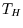and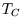occurs when the engine is reversible.

Answer here.

• Ex. 4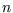moles of an ideal gas at temperature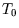are originally confined to half of an insulated container by a partition. The partition is removed without doing any work. What is the change in entropy?

Answer: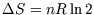. More details here.

• Ex. 5 An insulated container is originally divided in half by a partition, and each half is originally occupied bymoles of an different ideal gas at temperature. The partition is removed without doing any work. What is the change in entropy?

Answer: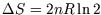. More details here.

References

• (Mandl 2.1)
• Bowley and Sánchez 2.7
• Adkins 5.5-6
• Zemansky 8.11-13

SubsectionsNext: 2.7 The Fundamental Thermodynamic Relation Previous: 2.5 Entropy
Judith McGovern 2004-03-17